# Representation of a compact group(2)

Letbe a compact group, letbe a Banach space and let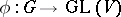be a representation. If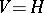is a Hilbert space andis a unitary operator for every, thenis called a unitary representation. There always is an equivalent norm infor whichis unitary.
Every irreducible unitary representation (cf. Irreducible representation) of a compact groupis finite-dimensional. Let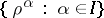be the family of all possible pairwise inequivalent irreducible unitary representations of the group. Every unitary representationofis an orthogonal sum of unique representations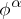,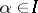, such thatis an orthogonal sum, possibly zero, of a set of representations equivalent to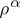.
Ifis finite, then the family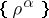is also finite and contains as many elements as there are distinct conjugacy classes in(moreover,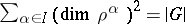). The problem of studying these representations (computing their characters, finding explicit realizations, etc.) is the subject of an extensive theory (cf. Finite group, representation of a).
Ifis a connected, simply-connected, compact Lie group and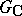is its complexification (cf. Complexification of a Lie group), then the description of the familyforamounts (by restricting the representations to) to the description of the family of all irreducible pairwise inequivalent finite-dimensional rational representations of the reductive algebraic group. The latter family, in turn, allows of a complete description by considering highest weights (cf. Representation with a highest weight vector).
In modern number theory and algebraic geometry one considers-adic representations of compact totally-disconnected groups (cf. , ).